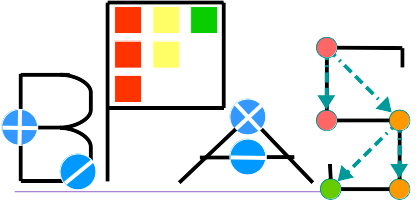## Installation Guide

### Requirements

- Linux systems, i.e. Ubuntu
- GCC version 6+ to compile CilkPlus programs
as well as C++11 features.
- CMake version 3.1+
- GMP library version 5.1.* or later with C++ support
- Python 2
- MPSolve version 3.1.4

### Building

Instructions can be found in the top-level README. In brief, unpack the tar file and perform the following:
``` mkdir build && cd build ccmake .. make make check make sanity-tests ## sanity tests make validate-tests ## testing against maple make test ## both sanity-tests and validate-tests make install ## optional; will copy library and headers to /usr ```

### Building a BPAS application with g++ compiler

- compiling:

 Flags Note -fcilkplus CilkPlus flag required -DLINUXINTEL64=1 Modpn 64-bit mode required

 Flags Note -lgmpxx -lgmp GMP library required -lcilkrts CilkPlus library required -lmodpnLINUXINTEL64 Modpn library required -lmps -lpthread MPSolve required -lbpas BPAS library required

### Real root isolation examples: examples.zip

We provide some examples, that is, the input files in "examples.zip", for univariate and multivariate real root isolation.

To run those examples:
- Put the "examples" folder under the same directory as "BPAS" folder
- cd BPAS/tests/RealRootIsolation
- make univariate (executing univariate cases) or make multivariate (executing multivariate cases)

## A Simple Example

`In main.cpp`:
```#include <bpas.h>

int main(int argc, char *argv[]) {
int d = 4095;
DUZP a(d+1), b(d+1);
// Assigning random coefficients to a and b
gmp_randclass r (gmp_randinit_default);
r.seed(time(NULL));
for (int i = 0; i <= d; ++i) {
a.setCoefficient(i, r.get_z_bits(d));
b.setCoefficient(i, r.get_z_bits(d));
}
/* Univariate Integer Polynomial Multiplication */
DUZP c = a * b;

/* Univeriate Real Root Isolation */
DUQP p;
p.setVariableName('x');
p = ((p + mpq_class(1)) << 2) - mpq_class(2);  // p := x^2-2
mpq_class width(1, 100);
Intervals boxes = p.realRootIsolate(width);
std::cout << "boxes -> " << boxes << std::endl;

/* Conversion Between Polynomial Classes */
SMQP q("(x+1)*y^4-2*x*y^2+3*x^2+1");
SparseUnivariatePolynomial<SMQP> s = q.convertToSUP('x');
SMQP z (s);
if (z != q) {
std::cout << z << " and " << q << " should not differ " << std::endl;
}

/* Symbolic Integration */
Symbol x_sym('x');
SparseUnivariatePolynomial<RationalNumber> x(x_sym); // identity
SparseUnivariatePolynomial<RationalNumber> f, g;
f.one(); f.setVariableName(x_sym);
g = RationalNumber(1) + RationalNumber(2)*x + (RationalNumber(2)*x)^2;
g ^= 4;
UnivariateRationalFunction<SparseUnivariatePolynomial<RationalNumber>, RationalNumber> h (f, g);
h.realSymbolicNumericIntegrate(53);

return 0;
}
```
`Its Makefile`:
```CILKPP  = g++
LIBARG  = -lbpas -lgmpxx -lgmp -lcilkrts -lmodpnLINUXINTEL64 -lmps -lpthread
COMPILEARG = -c -O2 -g -fcilkplus -DLINUXINTEL64=1
TARGET  = main

all: \$(TARGET)

\$(TARGET): \$(TARGET).o
\$(CILKPP) -o \$@ \$^ \$(LIBARG)

\$(TARGET).o: \$(TARGET).cpp
\$(CILKPP) \$(COMPILEARG) \$<
```

ORCCA Lab, Department of Computer Science, The University of Western Ontario, London, Ontario, Canada N6A 5B7